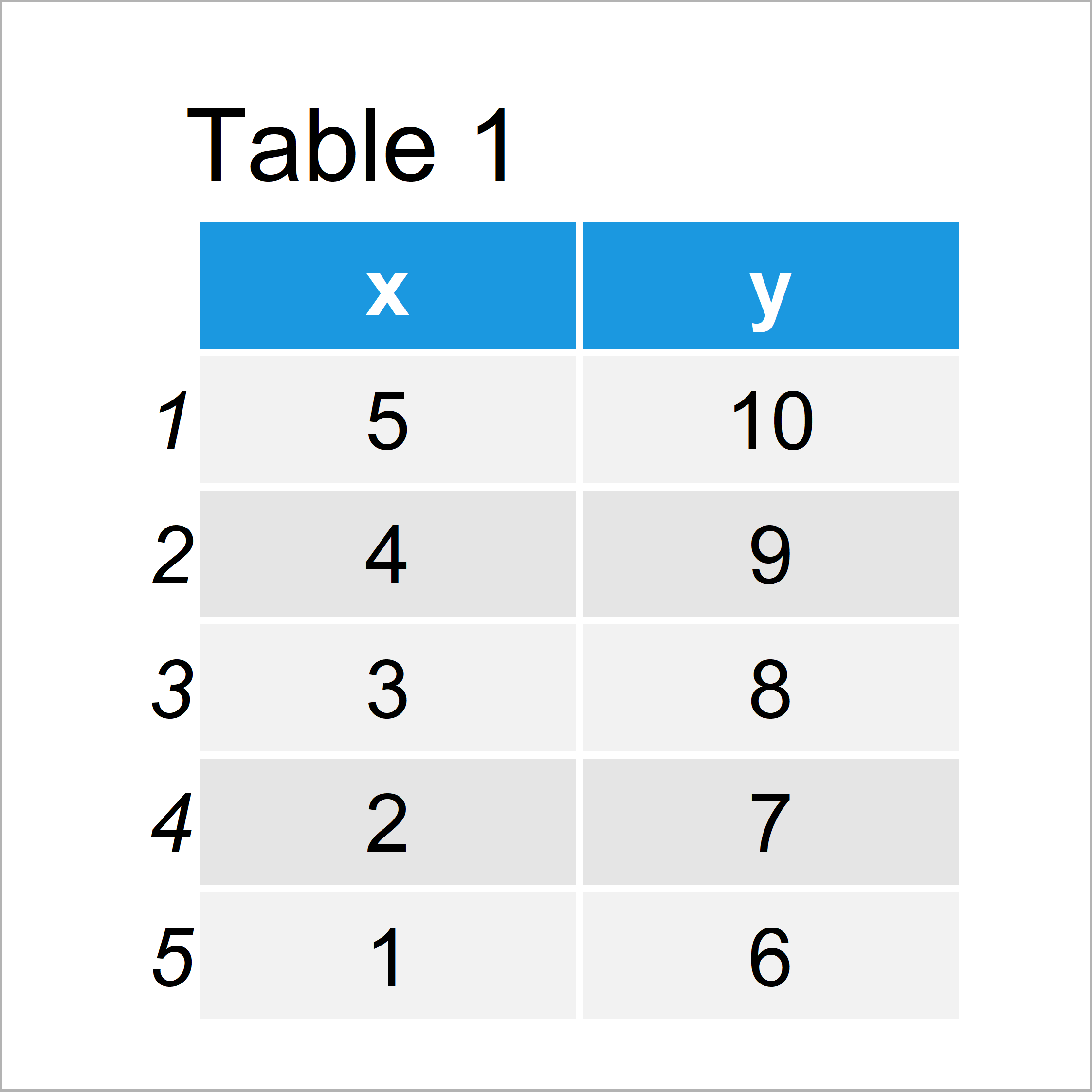# R ggplot2 Error: Mapping should be created with `aes()` or `aes_()`.

In this R tutorial you’ll learn how to debug the ggplot2 error message “Error: Mapping should be created with `aes()` or `aes_()`.”.

The content of the article is structured as follows:

It’s time to dive into the exemplifying R code…

## Example Data & Packages

First, let’s create some example data:

```data <- data.frame(x = 5:1, # Create example data y = 10:6) data # Print example data```The previous table illustrates the output of the RStudio console returned after running the previous syntax and shows that our exemplifying data is constituted of five rows and two columns with the names “x” and “y”.

For the examples of this tutorial, we also need to install and load the ggplot2 package.

```install.packages("ggplot2") # Install ggplot2 package library("ggplot2") # Load ggplot2 package```

Now, we are set up and can move on to the examples!

## Example 1: Reproduce the Error – Mapping should be created with `aes()` or `aes_()`.

This example demonstrates how to replicate the “Error: Mapping should be created with `aes()` or `aes_()`.” when using the ggplot2 package in the R programming language.

Have a look at the following R code:

```ggplot(data, aes(x, y) + # Reproduce error message geom_point()) # Error: Mapping should be created with `aes()` or `aes_()`.```

As you can see, the previous R code returned the error message “Error: Mapping should be created with `aes()` or `aes_()`.” to the RStudio console.

The reason for this is that we have put the last parenthesis at the wrong position (i.e. after geom_point).

So where should we put this parenthesis, and how can we debug this error message? That’s what I’ll explain next!

## Example 2: Debug the Error – Mapping should be created with `aes()` or `aes_()`.

In Example 2, I’ll show how to solve the problems with the “Error: Mapping should be created with `aes()` or `aes_()`.”.

For this we have to move the very las parenthesis one line upwards in front of the + sign.

Consider the R syntax below:

```ggplot(data, aes(x, y)) + # Fix error message geom_point()```As you can see, we have not received an error message. Instead, the graphic shown in Figure 1 has been created.

## Video, Further Resources & Summary

I have recently published a video on the Statistics Globe YouTube channel, which illustrates the R programming codes of this tutorial. You can find the video below:

Also, you could have a look at the related articles of this homepage.

In summary: In this R tutorial you have learned how to deal with the ggplot2 error message “Error: Mapping should be created with `aes()` or `aes_()`.”. Let me know in the comments, in case you have additional questions.

Subscribe to the Statistics Globe Newsletter

•